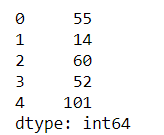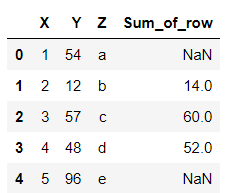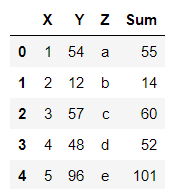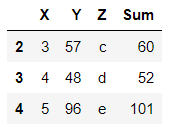# How to sum values of Pandas dataframe by rows?

• Last Updated : 26 Mar, 2021

While working on the python pandas module there may be a need, to sum up, the rows of a Dataframe. Below are the examples of summing the rows of a Dataframe. A Dataframe is a 2-dimensional data structure in form of a table with rows and columns. It can be created by loading the datasets from existing storage, storage can be SQL Database, CSV file, an Excel file, or from a python list or dictionary as well.

Pandas dataframe.sum() function returns the sum of the values for the requested axis.

Syntax: DataFrame.sum(axis)

Parameters:

• axis : {index (0), columns (1)}

Sum of each row:

`df.sum(axis=1)`

Example 1:

Summing all the rows of a Dataframe using the sum function and setting the axis value to 1 for summing up the row values and displaying the result as output.

## Python3

 `# importing pandas module as pd``import` `pandas as pd`` ` `# creating a dataframe using dictionary``df ``=` `pd.DataFrame({``'X'``:[``1``, ``2``, ``3``, ``4``, ``5``],``                   ``'Y'``:[``54``, ``12``, ``57``, ``48``, ``96``]})`` ` `# sum() method sums up the rows and columns of a dataframe``# axis = 1 sums up the rows``df ``=` `df.``sum``(axis ``=` `1``)``print``(df)`

Output :Sum of all the rows by index

Example 2:

Summing all the rows or some rows of the Dataframe as per requirement using loc function and the sum function and setting the axis to 1 for summing up rows. It sums up only the rows specified and puts NaN values in the remaining places.

## Python3

 `# importing pandas as pd``import` `pandas as pd`` ` `# creating the dataframe using pandas DataFrame``df ``=` `pd.DataFrame({``'X'``:[``1``, ``2``, ``3``, ``4``, ``5``],``                   ``'Y'``:[``54``, ``12``, ``57``, ``48``, ``96``],``                   ``'Z'``:[``'a'``, ``'b'``, ``'c'``, ``'d'``, ``'e'``]})`` ` `# df['column_name'] = df.loc[start_row_index:end_row_index,``# ['column1','column2']].sum(axis = 1)``# summing columns X and Y for row from 1 - 3``df[``'Sum_of_row'``] ``=` `df.loc[``1` `: ``3``,[``'X'` `, ``'Y'``]].``sum``(axis ``=` `1``)``print``(df)`

Output :Summing all rows from row 1 to 3

Example 3 :

Summing the rows using the eval function to evaluate the sum of the rows with the specified expression as a parameter.

## Python3

 `# importing pandas as pd``import` `pandas as pd`` ` `# creating the dataframe using pandas DataFrame``df ``=` `pd.DataFrame({``'X'``:[``1``, ``2``, ``3``, ``4``, ``5``],``                   ``'Y'``:[``54``, ``12``, ``57``, ``48``, ``96``],``                   ``'Z'``:[``'a'``, ``'b'``, ``'c'``, ``'d'``, ``'e'``]})`` ` `# eval('expression') calculates the sum of the specified columns of that row``df ``=` `df.``eval``(``'Sum = X + Y'``)``print``(df)`

Output :Sum of rows using the eval function

Example 4 :

Summing the rows using the eval function to evaluate the sum of the rows with specified rows using loc with the expression to calculate the sum as a parameter to eval function. It only returns the rows which are being specified in the loc and chops off the remaining.

## Python3

 `# importing pandas as pd``import` `pandas as pd`` ` `# creating the dataframe using pandas DataFrame``df ``=` `pd.DataFrame({``'X'``:[``1``, ``2``, ``3``, ``4``, ``5``],``                   ``'Y'``:[``54``, ``12``, ``57``, ``48``, ``96``],``                   ``'Z'``:[``'a'``, ``'b'``, ``'c'``, ``'d'``, ``'e'``]})`` ` `# eval('expression') calculates the sum``# of the specified columns of that row``# using loc for specified rows``df ``=` `df.loc[``2``:``4``].``eval``(``'Sum = X + Y'``)``display(df)`

Output :Summing specified rows only using eval

My Personal Notes arrow_drop_up